# 2016/05/28 - Apache Tuscany has been retired.

## For more information, please explore the Attic.Apache Tuscany > Home > SCA Overview > SCA Java > Java SCA Documentation Menu > Getting Started with Tuscany ( using the Command Line) User List | Dev List | Issue Tracker
General
SCA Java
SCA Java 1.x
SCA Java 2.x
Resources

## What this is about

This guide takes a command line based approach to getting started with Apache Tuscany Java SCA. For the Eclipse users amongst us there are other guides that show how to install the distribution into Eclipse or how to exploit Tuscany SCA Java using the Eclipse plugin that is has been built as part of the project. This article however just uses the downloaded Tuscany SCA Java distribution, Java, Maven or Ant and the command line. So if you want to feel close to the action this is where to start!

Tuscany ships with a number of samples. One of the most straightforward of these is the calculator sample. We will use this to make sure that you installation is working properly. As the name indicates, this example performs typical calculator operations. It is given two numbers and asked to perform an operation on them. Our calculator will handle add, subtract, multiply and divide.

By the end of this exercise you know how to develop, deploy and run the calculator application and how to reconfigure it to make it work for different environments.

## Setup Environment

First of all we will walk through running the calculator sample to make sure you installation is working correctly. Then we will walk through the steps taken to implement the calculator sample in the first place. Following this you should be familiar with how to build a basic SCA application that runs in a single, stand-alone, SCA runtime. The next step is to update the sample to use some of the other Tuscany SCA Java extensions. Finally we will use the calculator sample to show you how you can build a distributed Service Oriented Architecture using Tuscany SCA Java.

• Download the Tuscany Java SCA release.
From this page you can get binary and source releases for both windows and linux. For this exercise you will need the binary release for your preferred platform. You can use the source code release but you will have to use Maven at all stages.
• Download java
Java 5
• Download a build tool
Maven 2.0.7+
OR
Ant 1.7.0

## Run An Existing Calculator Appliction

Tuscan SCA provides a calculator sample with its binary distribution. You can find it in the samples/calculator directory. As shown below, this sample and every sample in Tuscany has a "readme" that explains how to run the sample, a *.png file that has shows how the SCA application looks like.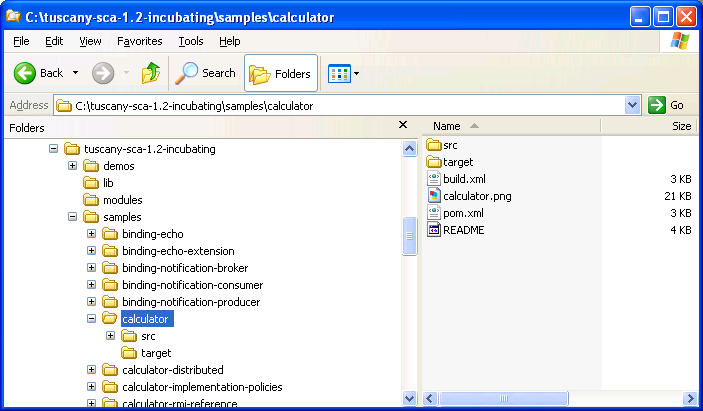Let's first run the sample before we go about building it. It is easy! Go to the directory ..\samples\calculator

```ant run
```

Alternatively if you want to run the sample directly from the command line try the following.

• if you are using Windows issue the command:
```java -cp ..\..\lib\tuscany-sca-manifest.jar;target\sample-calculator.jar calculator.CalculatorClient
```
• if you are using *nix issue the command:
```java -cp ../../lib/tuscany-sca-manifest.jar:target/sample-calculator.jar calculator.CalculatorClient
```

You should see the following result:

3 + 2=5.0
3 - 2=1.0
3 * 2=6.0
3 / 2=1.5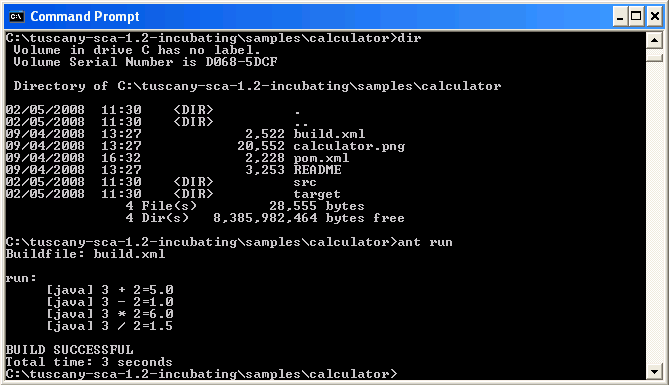If you are using the source distribution then we suggest you use Maven to build and run the calculator sample because the tuscany-sca-manifest.jar is not provided with the source distribution. This jar is part of the binary distribution and collects together all of the tuscany jars in one place so that the java command line is nice and short when running samples.

## Build The Calculator Sample In Java

This example illustrates how to define your application while staying focused on the business logic. It walks you through the steps of building the calculator sample. All connections between the components within the composite are local and described using Java interfaces.

Step 1 - Define what building blocks are needed: Think about how your application can be broken down into smaller functions/services. Each block is a logical unit of operation that can be used in the overall application. In this case, calculator application can be divided into five blocks: AddService block, SubstractService block, MultiplyService block and DivideService block and a main block that takes a request and routes it to the right operation. We have called this main block the CalculatorService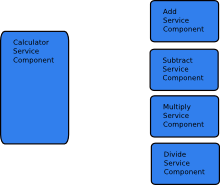Step 2 - Implement each block: Now that you have identified the blocks of functionality in your application, you are ready to create each block. In SCA the blocks of functionality are referred to as components so let's look at how we implement a component. We'll take the AddService component as our first example.

The AddService component will provide a service that adds two numbers together. The CalculatorService component uses the AddService component whenever it is asked to perform additions. If we were writing the AddService component in plain old Java we would start by describing a (Java) interface.

```public interface AddService {

double add(double n1, double n2);
}
```

Now, we provide an implementation of this interface.

```public class AddServiceImpl implements AddService {

public double add(double n1, double n2) {
return n1 + n2;
}
}
```

But wait! Aren't we writing an SCA component? It must be more complicated than plain old Java interface and implementation, right? Well, actually, an SCA component can just be plain old Java so we have just done all the coding we needed to implement the SCA AddService component. We can use SCA to expose the service that the AddService component provides over any of the supported bindings, for example, WebServices, JMS or RMI, without changing the AddService implementation.

Let's take a look at the CalculatorService component. This is interesting because it's going to call the AddService component. In the full application it will call the SubtractService, MultiplyService and DivideService components as well, but we will ignore these for the time being as they follow the same pattern as we will implement for the AddService component.

Again we will start by defining an interface because CalcultorService is itself providing an interface that others will call.

```public interface CalculatorService {

double add(double n1, double n2);
double subtract(double n1, double n2);
double multiply(double n1, double n2);
double divide(double n1, double n2);
}
```

Now we implement this interface.

```public class CalculatorServiceImpl implements CalculatorService {

private AddService addService;
private SubtractService subtractService;
private MultiplyService multiplyService;
private DivideService divideService;

@Reference
public void setAddService(AddService addService) {
this.addService = addService;
}

...set methods for the other attributes would go here

public double add(double n1, double n2) {
return addService.add(n1, n2);
}

...implementations of the other methods would go here
}
```

So we now have some components implemented using Java. Each component has a well defined interface, using Java interfaces in this case. Notice that these are just plain Java services with business logic and nothing else in them.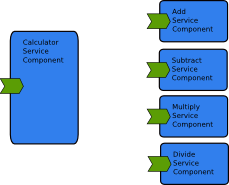## Assemble the Calculator Application

So far we have created the components. How do we actually run the calculator application? Well of course the java programmer in us wants to get down to it, write a mainline to connect our two components together and run then. We could still do that easily in this case.

```public class CalculatorClient {
public static void main(String[] args) throws Exception {

CalculatorServiceImpl calculatorService = new CalculatorServiceImpl();
AddService            addService        = new AddServiceImpl();
calculatorService.setAddService(addService);

System.out.println("3 + 2=" + calculatorService.add(3, 2));
// calls to other methods go here if we have implemented SubtractService, MultiplyService, DivideService
}
}
```

But this doesn't run using the Tuscany SCA runtime and extending this code to provide web services interfaces, for example, would be a little more complicated. What do we have to do to make it run in Tuscany where we get all things like web service support for free? Well, not much actually. First let's change the client to fire up the Tuscany SCA runtime before calling our components.

```public class CalculatorClient {
public static void main(String[] args) throws Exception {

SCADomain scaDomain = SCADomain.newInstance("Calculator.composite");
CalculatorService calculatorService = scaDomain.getService(CalculatorService.class, "CalculatorServiceComponent");

System.out.println("3 + 2=" + calculatorService.add(3, 2));
// calls to other methods go here if we have implemented SubtractService, MultiplyService, DivideService

scaDomain.close();
}
}
```

You can see that we start by using a static method on SCADomain to create a new instance of itself. The SCADomain is a concept in SCA that represents the boundary of an SCA system. This could be distributed across many processors. For now, lets concentrate on getting this working inside a single Java VM.

The parameter "Calculator.composite" refers to an XML file that tells SCA how the components in our calculator application are assembled into a working application. Here is the XML that's inside Calculator.composite.

```<composite xmlns="http://www.osoa.org/xmlns/sca/1.0"
name="Calculator">

<component name="CalculatorServiceComponent">
<implementation.java class="calculator.CalculatorServiceImpl"/>
<reference name="addService" target="AddServiceComponent" />
<!-- You can add references to SubtractComponent, MultiplyComponent and DivideComponent  -->
</component>

<component name="AddServiceComponent">
<implementation.java class="calculator.AddServiceImpl"/>
</component>

<!-- definitions of SubtractComponent, MultiplyComponent and DivideComponent -->

</composite>
```

In our case so far, we defined two components and we specify the Java implementation classes that Tuscany SCA needs to load to make them work in the .composite file. These are the classes we have just implemented.

Also note that the CalculatorServiceComponent has a reference named "addService". In the XML, this reference targets the AddServiceComponent. It is no coincidence that the reference name, "addService", matches the name of the addService field we created when we implemented CalculatorServiceImpl. The Tuscany SCA runtime parses the information from the XML composite file and uses it to build the objects and relationships that represent our calculator application. It first creates instances of AddServiceImpl and CalcualtorSreviceImpl. It then injects a reference to the AddServiceImpl object into the addService field in the CalculatorServiceImpl object. If you look back at how we implemented the CalculatorService you will see an @Reference annotation that tells SCA which fields are expecting to be set automatically by SCA. This is equivalent to this piece of code from our normal Java client.

```        CalculatorServiceImpl calculatorService = new CalculatorServiceImpl();
AddService            addService        = new AddServiceImpl();
calculatorService.setAddService(addService);
```

Once the composite file is loaded into the SCADomain our client code asks the SCADomain to give us a reference to the component called "CalculatorServiceComponent".

```        CalculatorService calculatorService = scaDomain.getService(CalculatorService.class, "CalculatorServiceComponent");
```

We can now use this reference as though we had created it ourselves, for example, from the CalculatorServiceImpl.add() method implementation.

```return addService.add(n1, n2);
```

The SCA specifications often describe SCA applications is diagrammatic form. This often helps give a quick overview of what components are part of an application and how they are wired together. If we draw a diagram of what we have build in the calculator sample we come up with something like.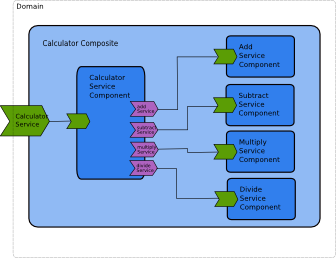You will notice that diagrams are provided with all of our samples. If you like to take a visual approach to things this may help you become quickly familiar with the components in the samples. Take a look at the ".png" files in the top level directory of each sample.

## Deploy the Calculator Application

The composite describes how SCA components are implemented and how they are assembled by wiring references to targets. This composite file has some dependencies, in this case the Java class and interface files that are used to implement the SCA components that it defines. The collection of composite files and other artifacts that are required to run an SCA application are collected together into one or more SCA contributions. A contribution can be a simple as a directory in a file system or could be packaged in, for example, a Jar file. SCA does not mandate any particular packing scheme. For our calculator sample you can imagine the contribution holding the calculator composite and all of its dependencies.

In fact if you look inside the jar file that the calculator sample produces, you will find the following

```calculator/AddService.class
calculator/AddServiceImpl.class
calculator/CalculatorClient.class
calculator/CalculatorService.class
calculator/CalculatorServiceImpl.class
calculator/DivideService.class
calculator/DivideServiceImpl.class
calculator/MultiplyService.class
calculator/MultiplyServiceImpl.class
calculator/SubtractService.class
calculator/SubtractServiceImpl.class
Calculator.composite
```

Which are all the artifacts that are required to run the calculator sample. We just need to add this contribution to the Tuscany SCA java runtime and then call the services that will be enabled.

The samples in Tuscany come with an Ant build.xml file that allows the sample files to be rebuilt so if you want to experiment with the sample code you can do so and then recompile it.

```ant compile
```

Once recompiled you can run it as before in the Running The Calculator Sample section, for example, we provide a run target in the Ant build.xml file so the calculator sample can also be run using.

```ant run
```

## Reconfigure The Calculator Application - Change Bindings

Looking back, the client code we have written to start the calculator application using the Tuscany SCA runtime is no longer than a normal Java client for the application. However we do now have the XML composite file that describes how our application is assembled.

This concept of assembly is a great advantage as our applications become more complex and we want to change them, reuse them, integrate them with other applications or just further develop them using a programming model consistent with all our other SCA applications. Regardless of what language is used to implement each of them.

For example, lets say our calculator sample is so poweful and popular that we want to put it on the company intranet and let other people access it as a service directly from their browser based Web2.0 applications. It's at this point we would normally start reaching for the text books to work out how to make this happen. As we have an XML file that describes our application it's easy in Tuscany SCA. The following should do the trick.

```<composite xmlns="http://www.osoa.org/xmlns/sca/1.0"
name="Calculator">

<service name="CalculatorService" promote="CalculatorServiceComponent/CalculatorService">
<interface.java interface="calculator.CalculatorService"/>
<binding.jsonrpc/>
</service>

<component name="CalculatorServiceComponent">
<implementation.java class="calculator.CalculatorServiceImpl"/>
<reference name="addService" target="AddServiceComponent" />
<!-- references to SubtractComponent, MultiplyComponent and DivideComponent  -->
</component>

<component name="AddServiceComponent">
<implementation.java class="calculator.AddServiceImpl"/>
</component>

<!-- definitions of SubtractComponent, MultiplyComponent and DivideComponent -->

</composite>
```

All we have done is added the <service> element which tells Tuscany SCA how to expose our CalculatorServiceComponent as a JSONRPC service. Note that we didn't have to change the Java code of our components. This is just a configuration change. The helloworld-jsonrpc sample shows a working example of the jsonrpc binding that you can use as an example to change the calculator application to use JSONRPC.

If we really wanted a SOAP/HTTP web service we can do that easily too.

```<composite xmlns="http://www.osoa.org/xmlns/sca/1.0"
name="Calculator">

<service name="CalculatorService" promote="CalculatorServiceComponent/CalculatorService">
<interface.java interface="calculator.CalculatorService"/>
<!-- ** Below we added info for json binding ** -->
<binding.jsonrpc/>
<binding.ws/>
</service>

<component name="CalculatorServiceComponent">
<implementation.java class="calculator.CalculatorServiceImpl"/>
<reference name="addService" target="AddServiceComponent" />
<!-- references to SubtractComponent, MultiplyComponent and DivideComponent  -->
</component>

<component name="AddServiceComponent">
<implementation.java class="calculator.AddServiceImpl"/>
</component>

<!-- definitions of SubtractComponent, MultiplyComponent and DivideComponent -->

</composite>
```

We have added binding.ws here so that your calculator service is available over JSONRPC and WebService bindings at the same time. The helloworld-ws-service and helloworld-ws-reference samples show you how to work with web services.

SCA allows other kinds of flexibility. We can rewire our components, for example, using a one of the remote bindings, like RMI, we could have the CalculatorServiceComponent running on one machine wired to a remote version of the application running on another machine. The calculator-rmi-service and calculator-rmi-reference samples show the RMI binding at work.

## Use Alternative Implementation Types

We could also introduce components implemented in different languages, for example, let's add the SubtractServiceComponent implemented in Ruby.

```<composite xmlns="http://www.osoa.org/xmlns/sca/1.0"
name="Calculator">

<component name="CalculatorServiceComponent">
<implementation.java class="calculator.CalculatorServiceImpl"/>
<reference name="addService" target="AddServiceComponent" />
<reference name="subtractService" target="SubtractServiceComponent" />
<!-- references to MultiplyComponent and DivideComponent  -->
</component>

<component name="AddServiceComponent">
<implementation.java class="calculator.AddServiceImpl"/>
</component>

<component name="SubtractServiceComponent">
<implementation.script script="calculator/SubtractServiceImpl.rb"/>
</component>

<!-- definitions of MultiplyComponent and DivideComponent -->

</composite>
```

Of course we need the Ruby code that implements the component.

```def subtract(n1, n2)
return n1 - n2
end
```

The Tuscany SCA runtime handles wiring Java components to Ruby components and performs any required data transformations. The calculator-script sample shows different script languages in use.

So, now that our application is described as an SCA assembly there are lots of possibilities as we further develop it and integrate it with other applications.